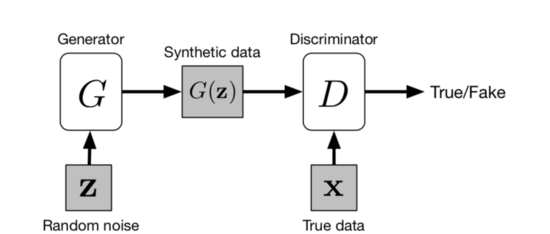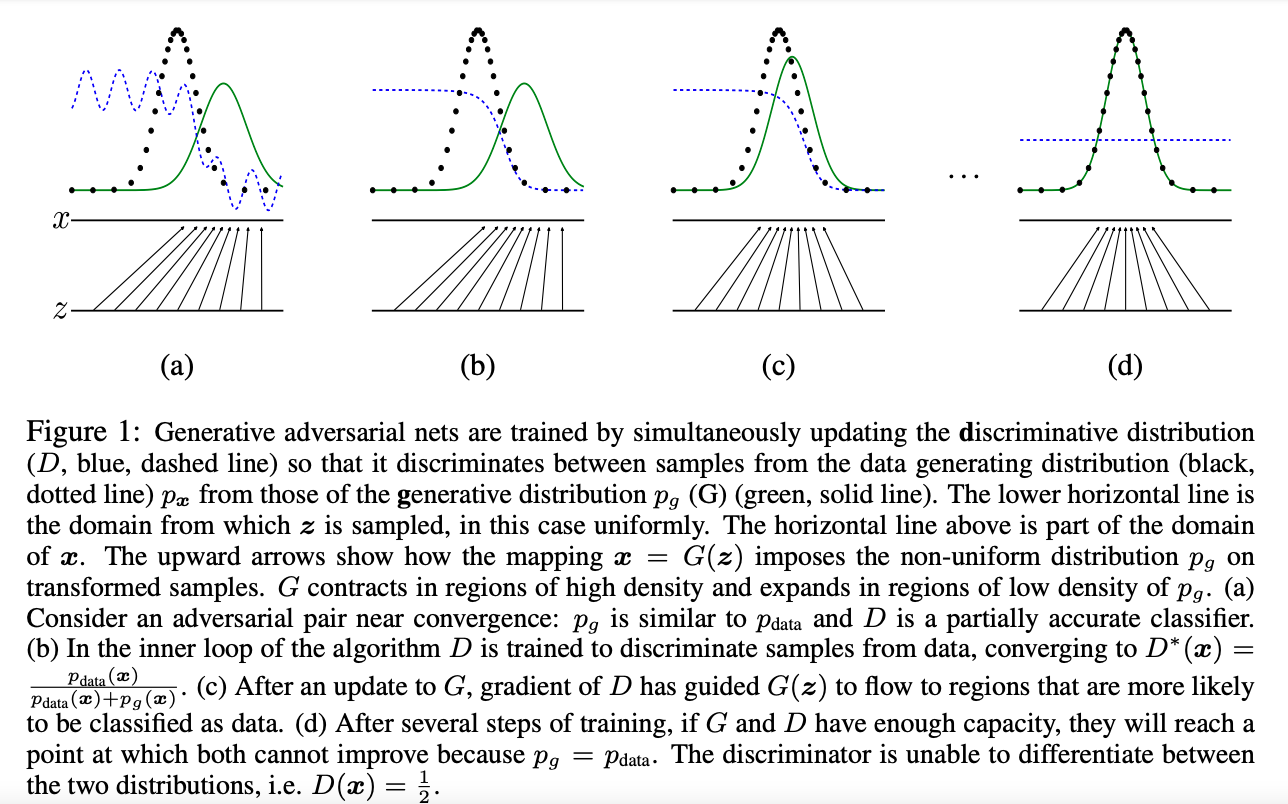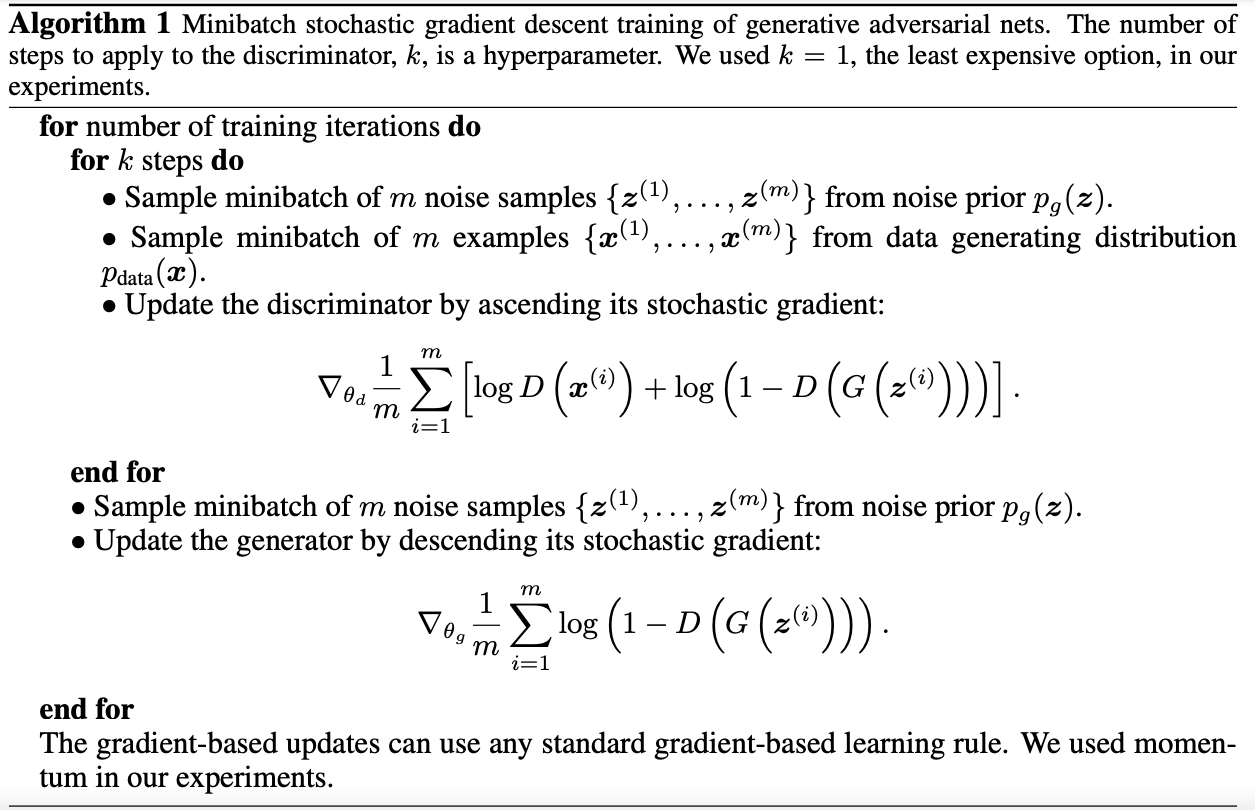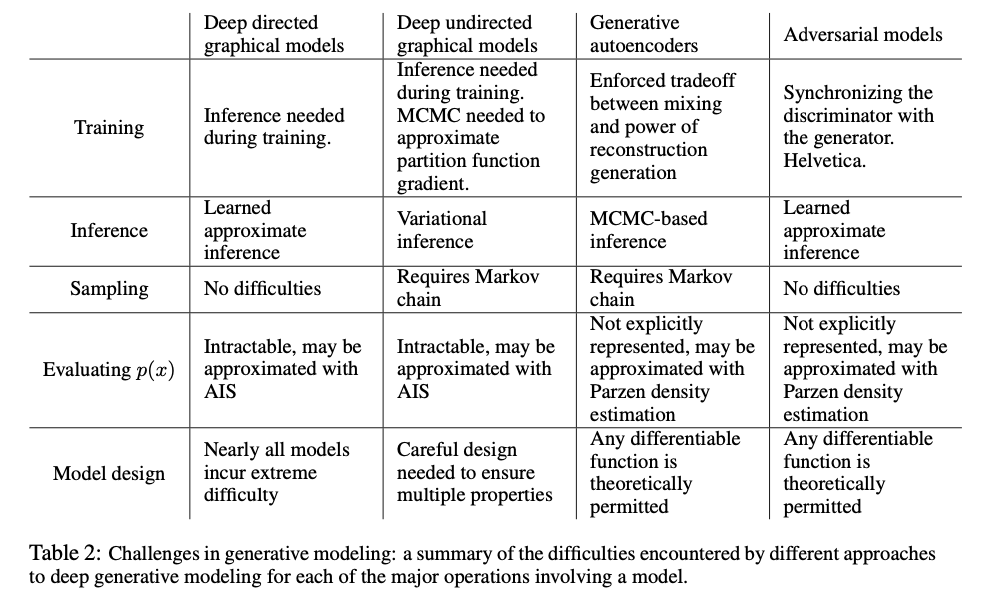## 问题

• 在极大似然估计和相关策略中出现的许多难以处理的概率计算的近似性
• 难以在生成环境中利用分段线性单元的优点

## 解决方案

adversarial nets是通过对抗过程估计生成模型的框架，同时训练两个模型，一个生成模型G捕获数据分布，一个判别模型D估计样本来自训练数据而不是G的概率。## Adversarial nets

Adversarial nets框架最直接的应用就是将生成模型$G$和判别模型$D$都配置成多层感知器。为了在数据$x$上学习生成模型G的分布$p_g$，我们定义了一个先验的输入噪声变量$p_z(z)$，然后将噪声变量到数据空间的映射表示为$G(z;θg)$，其中$G$是由多层感知器表示的微分函数。我们还定义了输出单个标量的多层感知器$D(x;θd)$$D(x)$代表$x$来自训练数据而不是由$p_g$生成的概率。我们训练判别模型$D$来最大化区分生成模型$G$产生的样本和训练样本，同时训练生成模型$G$来最小化$log(1-D(G(z)))$:

D和G玩了一个具有值函数$V(G,D)$的二人极大极小博弈,D要以最大可能判别出是生成数据，G要生成的数据与真实数据的差距尽可能的小,也就是在循环的过程中同时增强判别器D判别能力与提高生成器G的生成更接近真实数据的能力:

$\min _{G} \max _{D} V(D, G)=\mathbb{E}_{\boldsymbol{x} \sim p_{\text {data }}(\boldsymbol{x})}[\log D(\boldsymbol{x})]+\mathbb{E}_{\boldsymbol{z} \sim p_{\boldsymbol{z}}(\boldsymbol{z})}[\log (1-D(G(\boldsymbol{z})))]$

$\min _{G} \max _{D}$的理解：## Theoretical Results

GAN算法流程

• 第一步我们训练$D$$D$是希望$V(G, D)$越大越好，所以是加上梯度(ascending)。
• 第二步训练$G$时，$V(G, D)$越小越好，所以是减去梯度(descending)。
• 整个训练过程交替进行。• 命题 1：对于固定的G，最优鉴别器D为$D_{G}^{*}(\boldsymbol{x})=\frac{p_{\text {data }}(\boldsymbol{x})}{p_{\text {data }}(\boldsymbol{x})+p_{g}(\boldsymbol{x})}$

• 定理 1：当且仅当pg = pdata时，得到虚拟训练准则C(G)的全局最小值。此时，C(G)等于- log4。

• 命题 2: 当$G$$D$有足够的容量时, 在给定$G$时,$D$能达到最优值,而$p_g$也能得到更新,以提升$G$

$\mathbb{E}_{\boldsymbol{x} \sim p_{\text {data }}}\left[\log D_{G}^{*}(\boldsymbol{x})\right]+\mathbb{E}_{\boldsymbol{x} \sim p_{g}}\left[\log \left(1-D_{G}^{*}(\boldsymbol{x})\right)\right]$

## 对比其他建模方案## 优缺点

• 模型只用到了反向传播,而不需要马尔科夫链
• 训练时不需要对隐变量做推断
• 可以将多种函数合并到模型中
• G的参数更新不是直接来自数据样本,而是使用来自D的反向传播

• 可解释性差,生成模型的分布$p_g(G)$没有显式的表达
• 比较难训练,$D$$G$之间需要很好的同步,例如$D$更新$k$次而$G$更新一次

## Conclusion and Future Work

1.CGAN，即根据给定的条件和随机分布，生成特定的数据。
2.通过训练一个给定x，预测z的辅助网络，用于样本之间的相似度检测。
3.可以训练一个shared model，给定任意子条件和随机分布，生成该条件对应的样本。
4.半监督学习：当训练数据有限时，可以使用discriminator的特征或者G网络来提升分类器的性能。
5.在训练的过程中，如果可以确定一个更好的z的分布，则训练速度和模型性能都会大大提升。